# Mirrors and LensesPage 4

#### WATCH ALL SLIDES

At sunsets, light rays from the sun are bent as they pass into the atmosphere

It is a gradual bend because the light passes through layers of the atmosphere, and each layer has a slightly different index of refraction

The Sun is seen to be above the horizon even after it has fallen below

Slide 26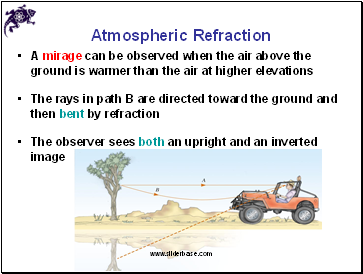Atmospheric Refraction

A mirage can be observed when the air above the ground is warmer than the air at higher elevations

The rays in path B are directed toward the ground and then bent by refraction

The observer sees both an upright and an inverted image

Slide 27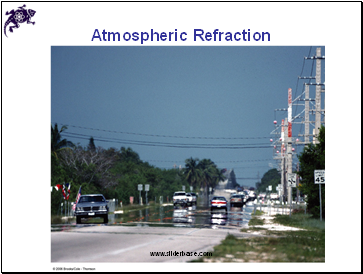Atmospheric Refraction

Slide 28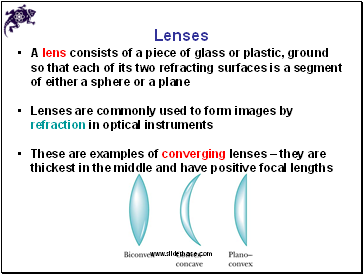## Lenses

A lens consists of a piece of glass or plastic, ground so that each of its two refracting surfaces is a segment of either a sphere or a plane

Lenses are commonly used to form images by refraction in optical instruments

These are examples of converging lenses – they are thickest in the middle and have positive focal lengths

Slide 29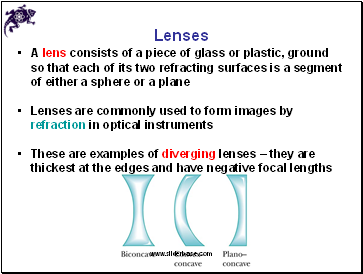Lenses

A lens consists of a piece of glass or plastic, ground so that each of its two refracting surfaces is a segment of either a sphere or a plane

Lenses are commonly used to form images by refraction in optical instruments

These are examples of diverging lenses – they are thickest at the edges and have negative focal lengths

Slide 30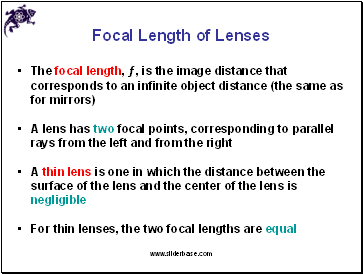## Focal Length of Lenses

The focal length, ƒ, is the image distance that corresponds to an infinite object distance (the same as for mirrors)

A lens has two focal points, corresponding to parallel rays from the left and from the right

A thin lens is one in which the distance between the surface of the lens and the center of the lens is negligible

For thin lenses, the two focal lengths are equal

Slide 31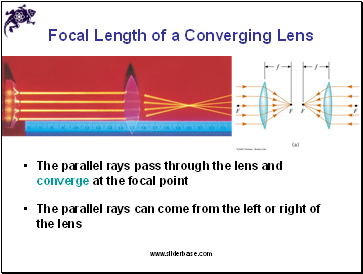Focal Length of a Converging Lens

The parallel rays pass through the lens and converge at the focal point

The parallel rays can come from the left or right of the lens

Slide 32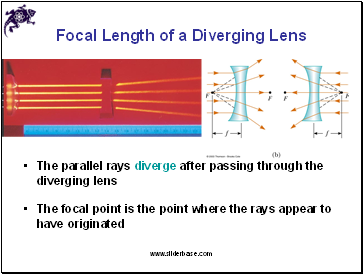Focal Length of a Diverging Lens

The parallel rays diverge after passing through the diverging lens

The focal point is the point where the rays appear to have originated

Slide 33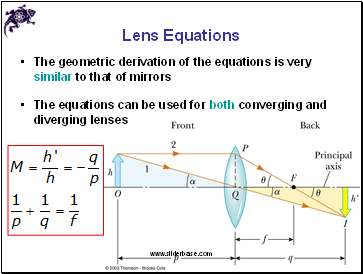## Lens Equations

The geometric derivation of the equations is very similar to that of mirrors

Go to page:
1  2  3  4  5  6  7